# PIL

## PIL Grammar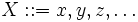$X ::= x,y,z,\dots$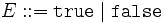$E ::= \texttt{true} \mid \texttt{false}$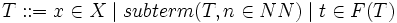$T ::= x \in X \mid subterm(T,n \in NN) \mid t \in F(T)$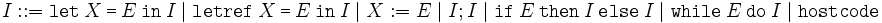$I ::= \texttt{let}~X~\texttt{=}~E~\texttt{in}~I \mid \texttt{letref}~X~\texttt{=}~E~\texttt{in}~I \mid X := E \mid I ; I \mid \texttt{if}~E~\texttt{then}~I~\texttt{else}~I \mid \texttt{while}~E~\texttt{do}~I \mid \texttt{hostcode}$

## PIL Semantics

Big-step, call-by-value semantics. ρ and σ range over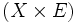$(X \times E)$.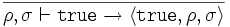$\frac{}{\rho,\sigma \vdash \texttt{true} \rightarrow \langle \texttt{true},\rho,\sigma \rangle}$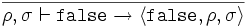$\frac{}{\rho,\sigma \vdash \texttt{false} \rightarrow \langle \texttt{false},\rho,\sigma \rangle}$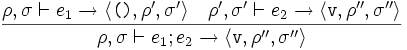$\frac{\rho,\sigma \vdash e_1 \rightarrow \langle \texttt{()},\rho',\sigma' \rangle \quad \rho',\sigma' \vdash e_2 \rightarrow \langle \texttt{v},\rho'',\sigma'' \rangle} {\rho,\sigma \vdash e_1 ; e_2 \rightarrow \langle \texttt{v},\rho'',\sigma'' \rangle}$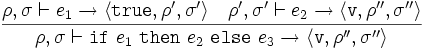$\frac{\rho,\sigma \vdash e_1 \rightarrow \langle \texttt{true},\rho',\sigma' \rangle \quad \rho',\sigma' \vdash e_2 \rightarrow \langle \texttt{v},\rho'',\sigma'' \rangle} {\rho,\sigma \vdash \texttt{if}~e_1~\texttt{then}~e_2~\texttt{else}~e_3 \rightarrow \langle \texttt{v},\rho'',\sigma'' \rangle}$$\frac{\rho,\sigma \vdash e_1 \rightarrow \langle \texttt{false},\rho',\sigma' \rangle \quad \rho',\sigma' \vdash e_3 \rightarrow \langle \texttt{v},\rho'',\sigma'' \rangle} {\rho,\sigma \vdash \texttt{if}~e_1~\texttt{then}~e_2~\texttt{else}~e_3 \rightarrow \langle \texttt{v},\rho'',\sigma'' \rangle}$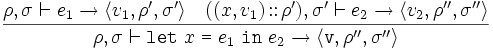$\frac{\rho,\sigma \vdash e_1 \rightarrow \langle v_1,\rho',\sigma' \rangle \quad ((x,v_1)\!::\!\rho'),\sigma' \vdash e_2 \rightarrow \langle v_2,\rho'',\sigma'' \rangle} {\rho,\sigma \vdash \texttt{let}~x~\texttt{=}~e_1~\texttt{in}~e_2 \rightarrow \langle \texttt{v},\rho'',\sigma'' \rangle}$